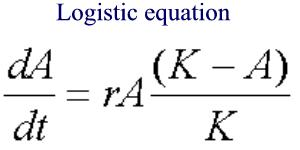|

# Equilibrium, Stability, and Behavior over time

#### Equilibrium, Stability, Behavior Over Time, Linear Process, and Time Delays

Equilibrium and Stability

To understand feedback processes it is important to understand the concepts of equilibrium and stability. Negative feedback loops are linked to stable equilibrium states and positive feedback loops are linked to unstable equilibrium. The stability of a system is intimately connected to its equilibrium state. If a system in equilibrium is disturbed slightly, then if it is stable it tends to return to or oscillate about its original equilibrium state. An unstable system tends to continue to move away from its original equilibrium state when perturbed from it. The figure at left shows a red ball in the bottom of a bowl, on top of an inverted bowl, or on a flat surface corresponding to stable, unstable, and neutral equilibrium states respectively.

Behavior over time (temporal behavior) There are many situations where the static balance (equilibrium) of a system is of central interest: for example in studies of geologic structures, bridges, or buildings. Modeling such systems and thinking in terms of systems can be extremely helpful in our understanding of how different components of such systems are interconnected. However, for our discussion regarding system dynamics and systems thinking our primary focus will be to understand the temporal behavior of dynamic systems. Understanding a system's expected behavior over time is a primary objective of system modeling and system dynamics. The graph below is an example of S-shaped growth obtained for a system that obeys the logistic equation (see footnote to the page). The growth of the system starts out exponentially until it becomes so large that its growth is limited. This model describes flowers growing in a field of limited size (800 sq. meters). Many think that World population will follow this sort of growth. The point here is not this specific example but to emphasize the importance of understanding the expected behavior of a system over time.

Linear Systems & Growth We refer to systems without loop structure or feedbacks as linear in that a cause has an effect, but the effect does not feedback and alter the cause. An example is a simple bucket filling with water. In reality someone will be watching the bucket fill and when it gets to the top they will turn off the faucet. This "feedback between water content and flow rate is a loop which would make the more realistic situation a non-linear system. The graph shows linear growth with two scenarios for filling the bucket with constant flow. Which line (A or B) corresponds to the greatest flow rate? From the graph, estimate this largest flow rate in gallons per minute.

• Linear thought is useful at times and appropriate for some situations.
• What does it mean when one says that "linear thought is a no-Karma thought process"?
• Thinking in terms of interconnectedness and loop structure is very important for understanding systems.
• The famous naturalist John Muir once said-- "When one tugs at a single thing in nature he finds it hitched to the rest of the universe"
Time Delays Between Cause and Effect

Understanding the dynamic behavior of systems requires the realization that there may be a significant delay between cause and effect.

In the figure a graph is shown for two hypothetical containers of cold (0 °C)water set in a 100 °C oven.

Can you tell which container of water is largest?

Why or why not?

Perturbations are small changes that disturb a system from its equilibrium state.

There is an inherent time delay between the time the perturbation is applied and when the new state is achieved.

Assuming that the graph applies to the figure below, what happened to cause the change in temperature shown in the graph?

#### FootnoteHere A is a variable (like area) and r and K are constants.